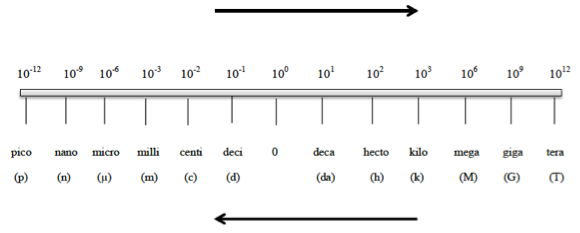# Problem: Use the prefix multipliers to express each of the following measurements without exponents.1.5 x 103 g

###### FREE Expert Solution

Metric Prefixes are “labels” that can be placed in front of base units. Whenever using the metric prefix chart, remember that “1” is associated with the metric prefixConversion factor needed:

1 kg = 103 g###### Problem Details

Use the prefix multipliers to express each of the following measurements without exponents.

1.5 x 103 g